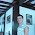## Sunday, February 22, 2009

### Problem 253: Triangle, Centroid, Vertices, Distances, Squares

Proposed ProblemSee complete Problem 253 at:
gogeometry.com/problem/p253_triangle_centroid_squares.htm

Level: High School, SAT Prep, College geometry

Post your solutions or ideas in the comments.

1.da=2ma/3; db=2mb/3; dc=2mc/3 and the mediane's theorem for ma, mb, mc.

2.Just to complete the earlier comment:
c^2+a^2-b^2/2=2*9/4*d(b)^2
b^2+c^2-a^2/2=2*9/4*d(a)^2
b^2+a^2-c^2/2=2*9/4*d(c)^2